You may also likeTriangle Midpoints

You are only given the three midpoints of the sides of a triangle. How can you construct the original triangle?Pareq Exists

Prove that, given any three parallel lines, an equilateral triangle always exists with one vertex on each of the three lines.The Medieval Octagon

Medieval stonemasons used a method to construct octagons using ruler and compasses... Is the octagon regular? Proof please.

Folding Fractions

Age 14 to 16Challenge Level

You may wish to look at Folding squares before trying this problem.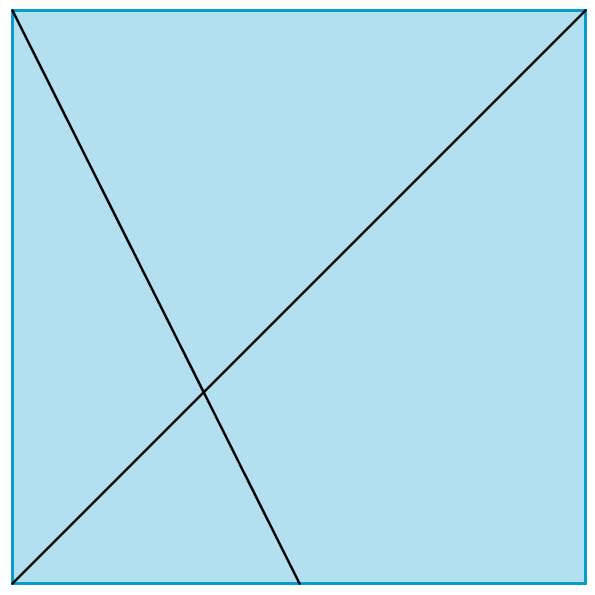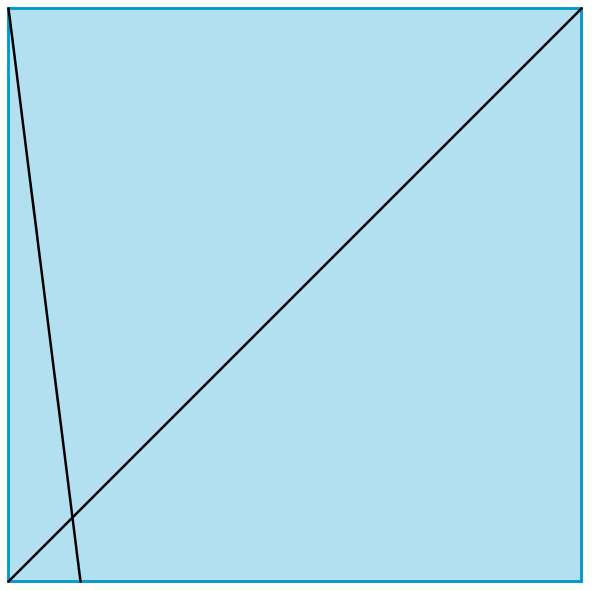You can divide the long diagonal of a square into different fractions by folding. In the first image the second fold joins a corner of the square to the midpoint of the opposite side. In the second image the second fold joins a corner of the square to a point $\frac{1}{8}$ of the way along the opposite side. This problem is about the fractions of the long diagonal of a square which you can construct in this way. To start with, we shall only consider points on the side of the square which can easily be found by folding. That is, $\frac{1}{2}$s or $\frac{1}{4}$s or $\frac{1}{8}$s and so on.
Investigate the fractions of the long diagonal of a square that can be created in the way described above. Here are some examples to think about: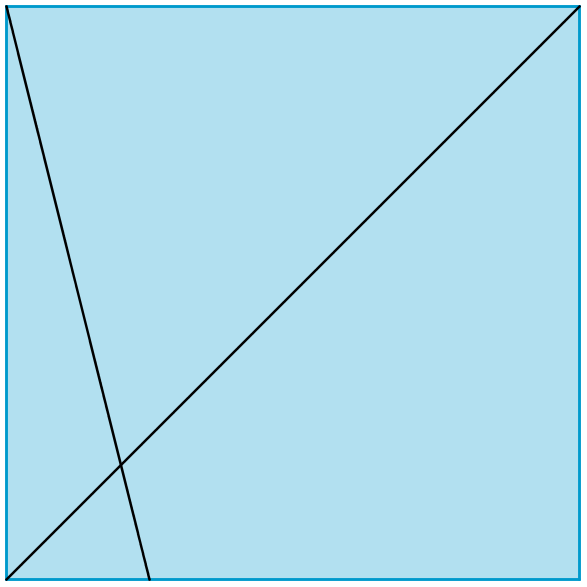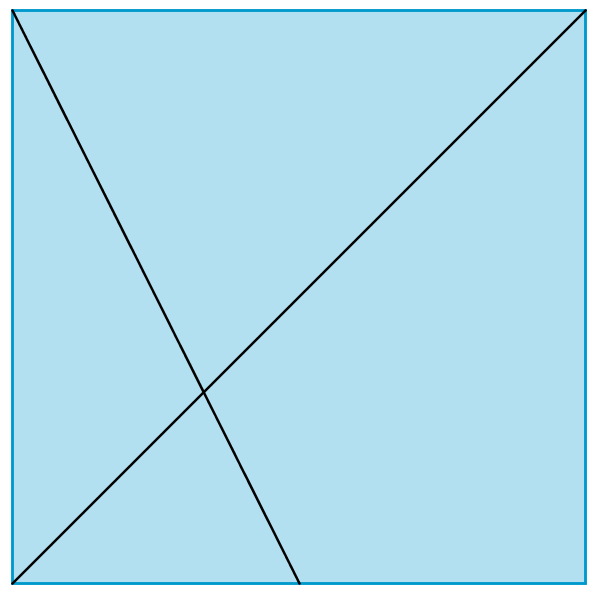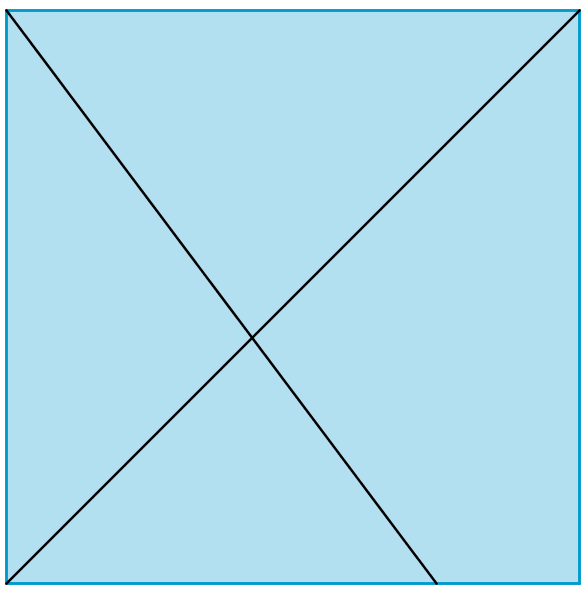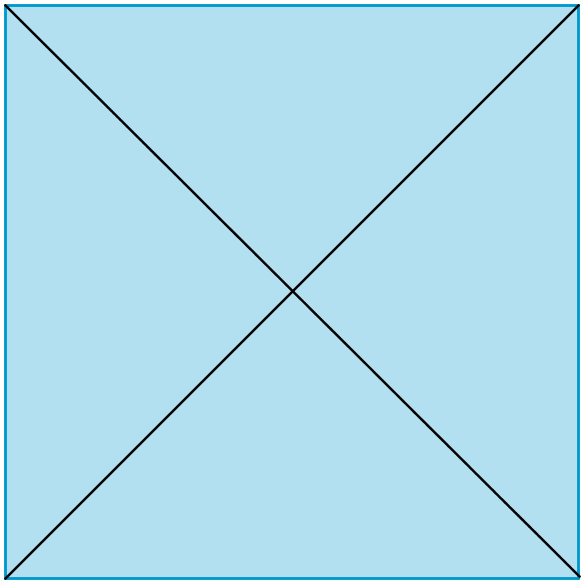...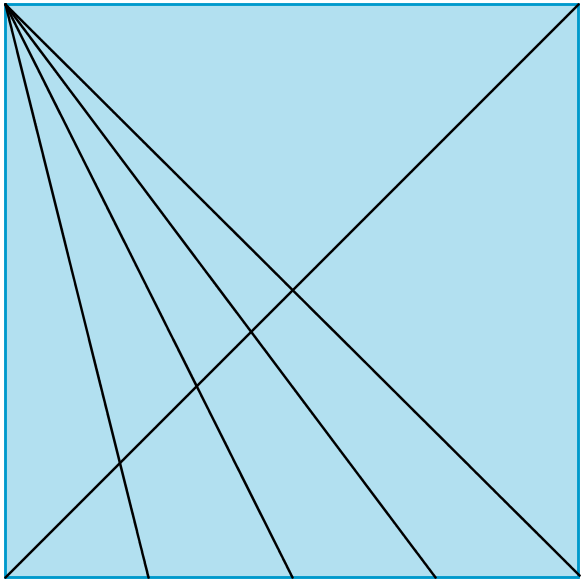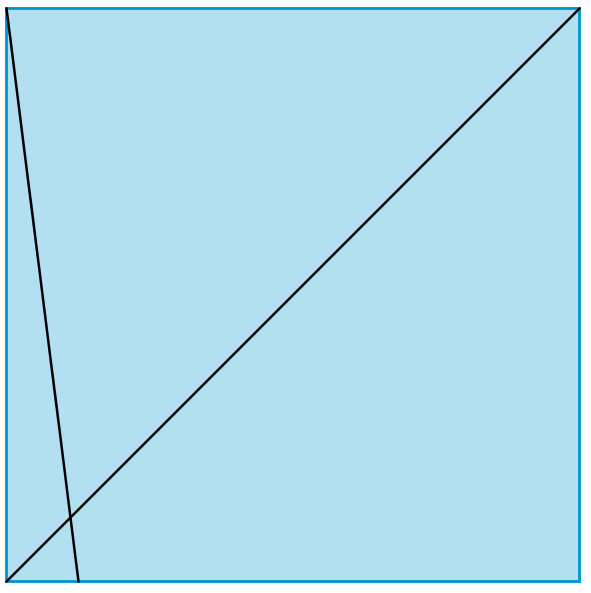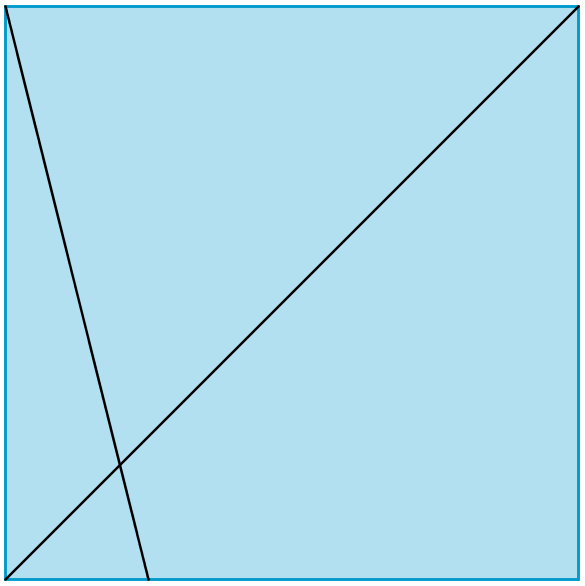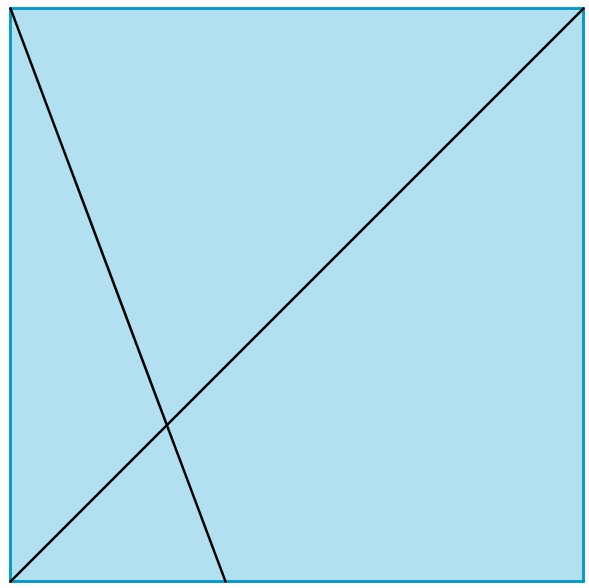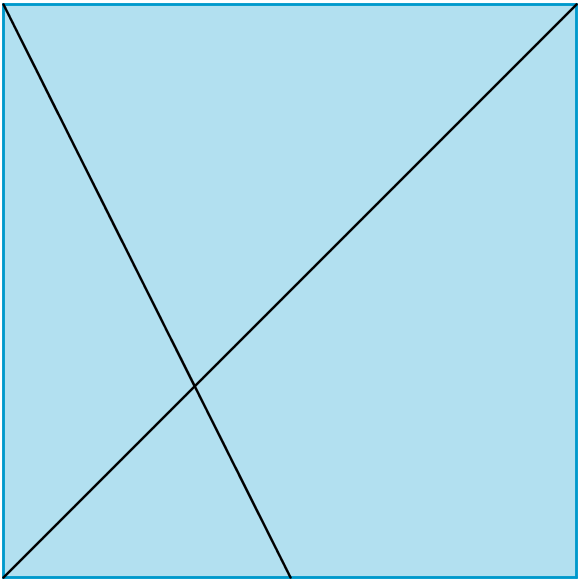...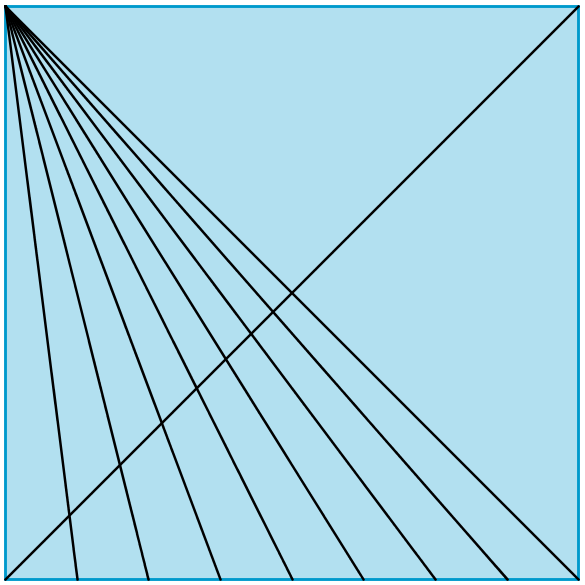Can you extend the findings and make generalisations?